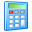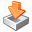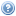Widgets# Deep Drawing Force Calculator

Calculate the required force to deep draw a piece of sheet metal based on the drawing ratio, sheet thickness, and the ultimate tensile strength of the material. The drawing ratio is a measure of the severity of the drawing operation and is the ratio of the initial blank diameter to the punch diameter. For a given material, the limiting drawing ratio (LDR) is a measure of that material’s deep drawability and is calculated from the largest blank that can be completely deep drawn for a given punch diameter. The amount of draw can also be represented as the percent reduction of the blank diameter. The calculated tonnage can be used for the selection of a machine to perform the deep drawing operation. Learn more about Deep Drawing.

## Deep Drawing Force Calculator

Sheet thickness (in):